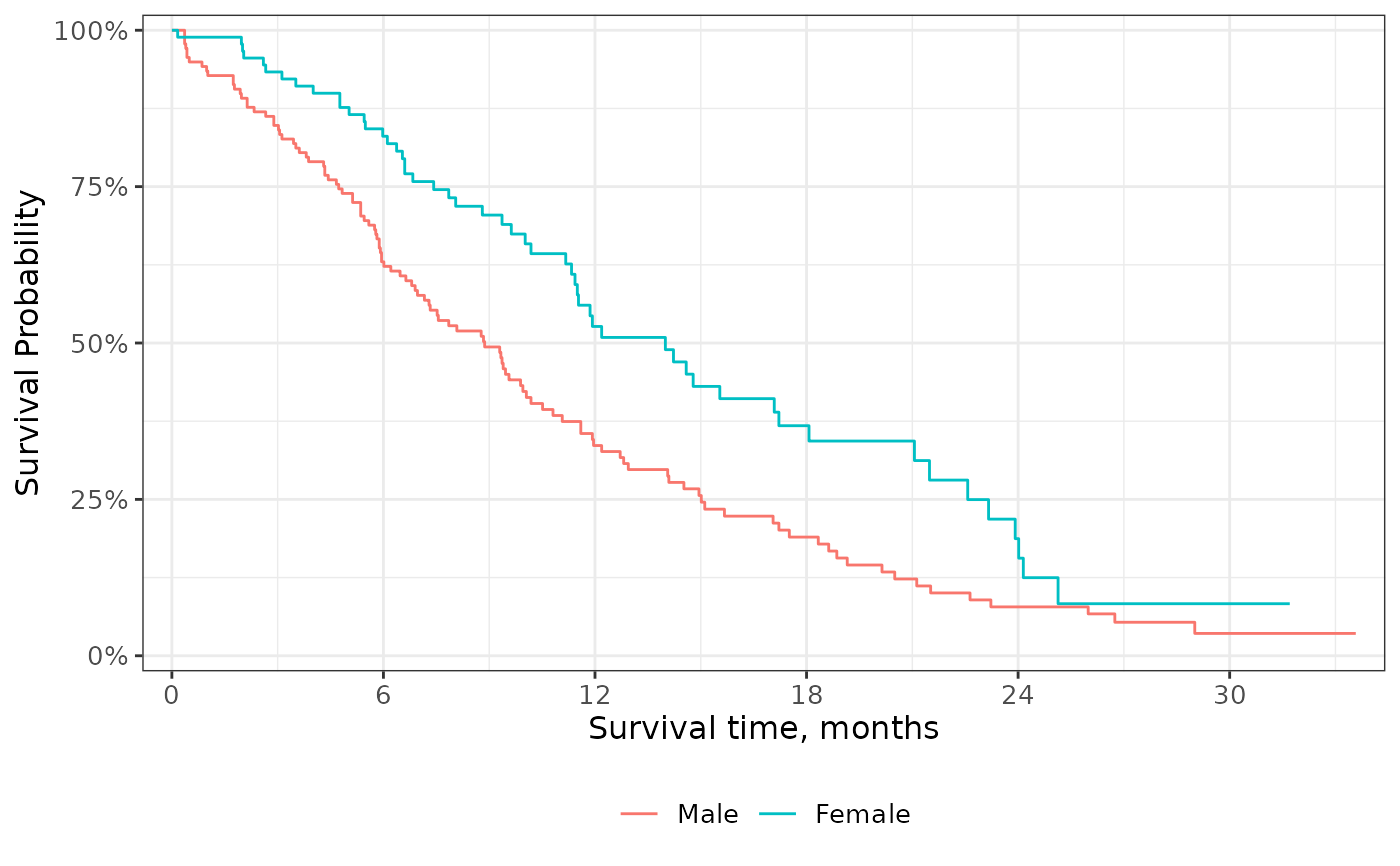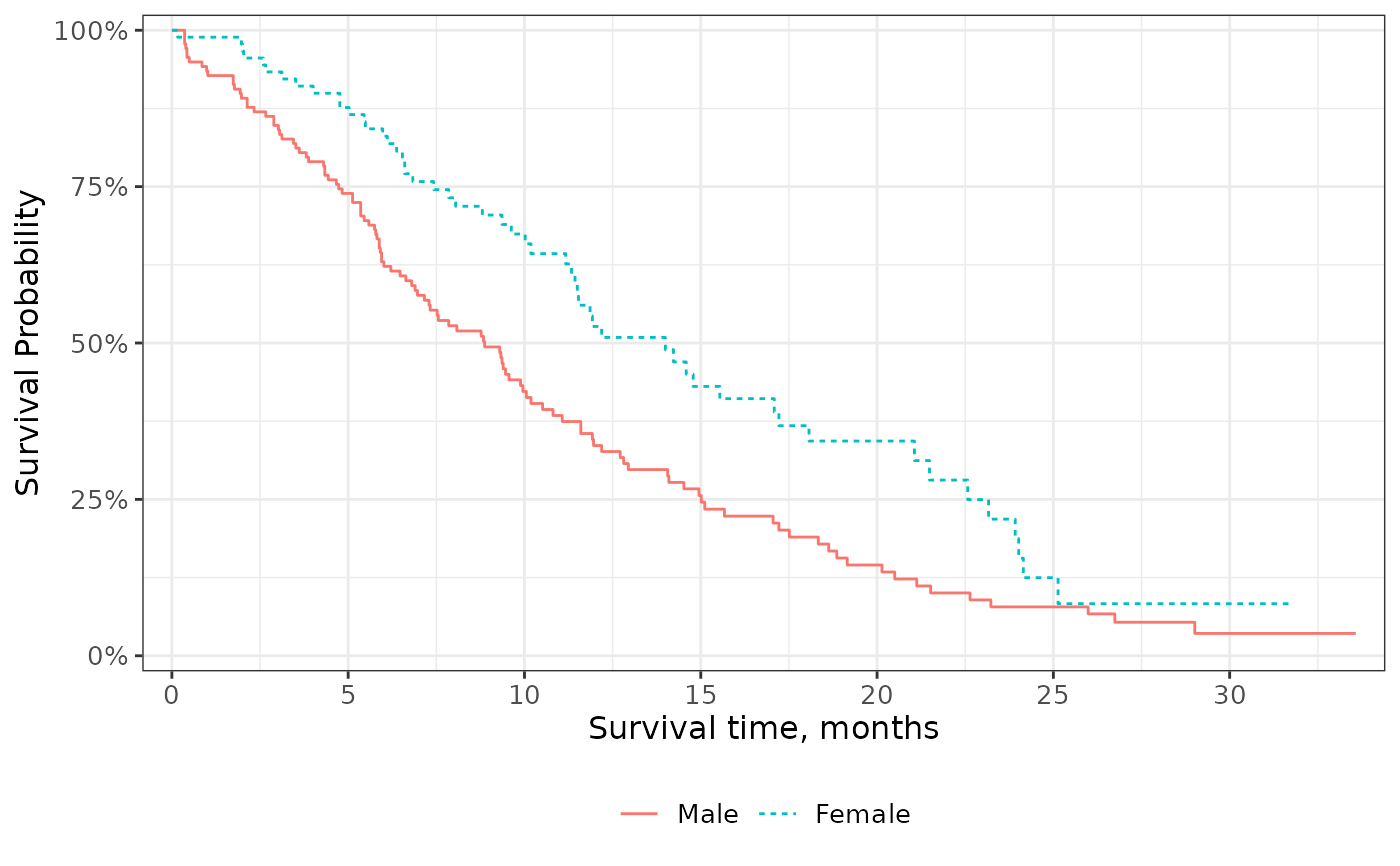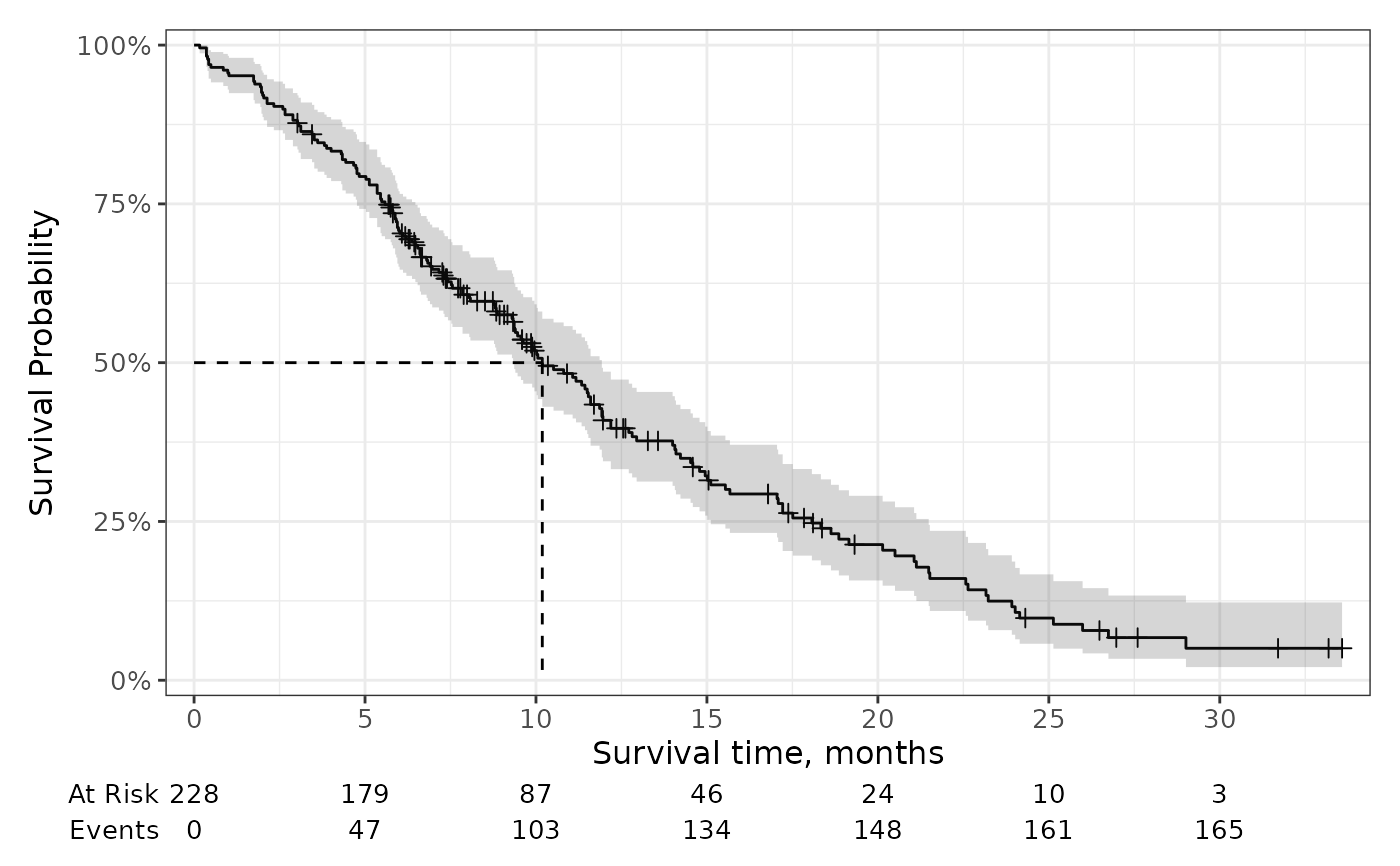Plot survival probabilities (and other transformations) using the results from survfit2() or survival::survfit(); although, the former is recommend to have the best experience with the ggsurvfit package.

## Usage

ggsurvfit(
x,
type = "survival",
linetype_aes = FALSE,
theme = theme_ggsurvfit_default(),
...
)

## Arguments

x

a 'survfit' object created with survfit2()

type

type of statistic to report. Available for Kaplan-Meier estimates only. Default is "survival". Must be one of the following:

 type transformation "survival" x "risk" 1 - x "cumhaz" -log(x)
linetype_aes

logical indicating whether to add ggplot2::aes(linetype = strata) to the ggplot2::geom_step() call. When strata are present, the resulting figure will be a mix a various line types for each stratum.

theme

a survfit theme. Default is theme_ggsurvfit_default()

...

arguments passed to ggplot2::geom_step(...), e.g. size = 2

a ggplot2 figure

## Details

This function creates a ggplot figure from the 'survfit' object. To better understand how to modify the figure, review the simplified code used internally:

survfit2(Surv(time, status) ~ sex, data = df_lung) %>%
tidy_survfit() %>%
ggplot(aes(x = time, y = estimate,
min = conf.low, ymax = conf.low,
color = strata, fill = strata)) +
geom_step()

Visit the gallery for examples modifying the default figures

## Examples

# Default publication ready plot
survfit2(Surv(time, status) ~ sex, data = df_lung) %>%
ggsurvfit()# Changing statistic type
survfit2(Surv(time, status) ~ sex, data = df_lung) %>%
ggsurvfit(type = "cumhaz")# Configuring KM line type to vary by strata
survfit2(Surv(time, status) ~ sex, data = df_lung) %>%
ggsurvfit(linetype_aes = TRUE)# Customizing the plot to your needs
survfit2(Surv(time, status) ~ 1, data = df_lung) %>%
ggsurvfit() +